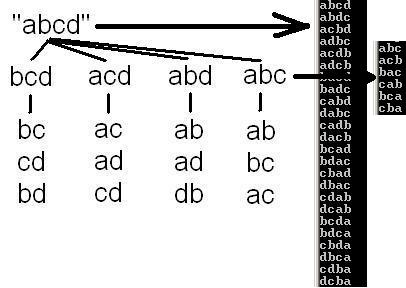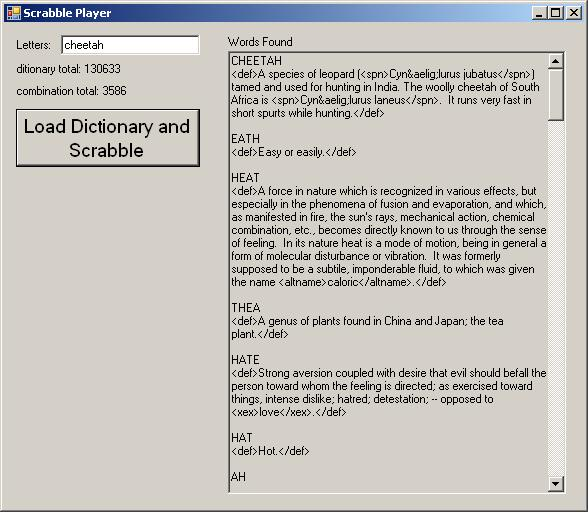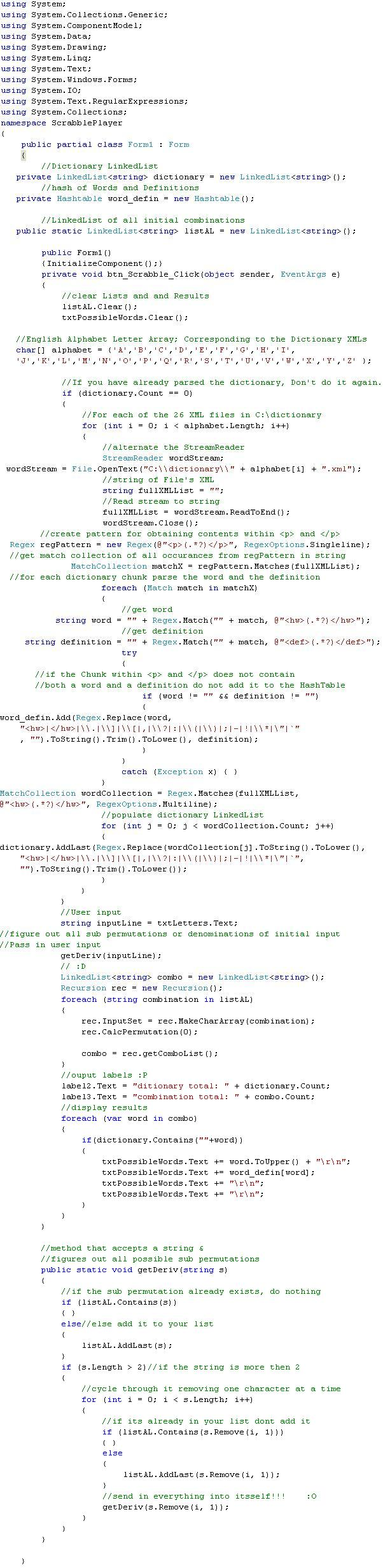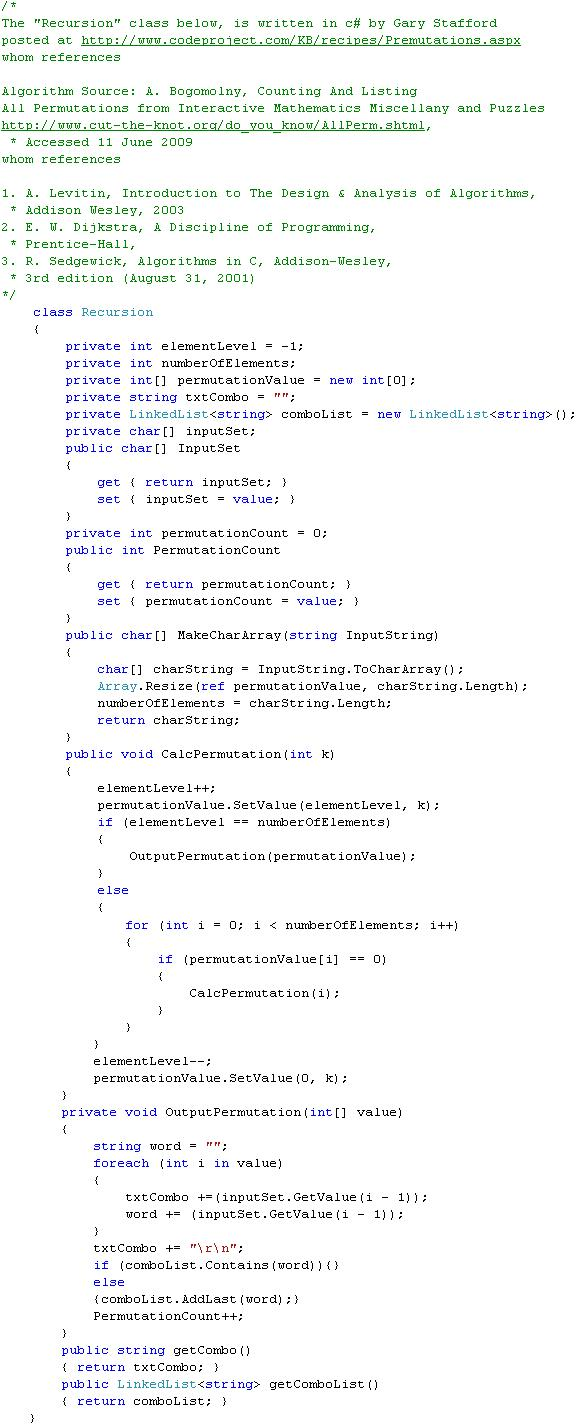# C#: Scrabble Player; Part 2 – Find all permutations of a string, parse a dictionary, cheat at Scrabble.

This an extension of a post from before.

C#: Find all permutations of a string, parse a dictionary, cheat at Scrabble.

ScrabblePlayer is a combination of my own joyous curiosity and an accumulation of my previous posts.  It is not built for performance.

This program accepts an input of English Letters from the user.  It then, figures out all possible permutations of the characters(letters), as well as, all permutations of sub-combinations down to a length of two.

Example: User inputs the word “abcd” ;

sub-combinations and -> permutations of input “abcdeach set has its own permutations recurviely.

1.) abcd : 4 characters, 24 permutations; (1*2*3*4)
2.) abc  : 3 characters, 6  permutations; (1*2*3)
3.) ab   : 2 characters, 2  permutations; (1*2)

Next, ScrabblePlayer parses a set of XML files which contain Websters Dictionary content.  The parsing populates both a LinkedList and a HashTable.  LinkedList<string> is set to the word, HashTable<string, string> is set to word as Key, and definition as Value.

After a LinkedList of both the accumulated permutations and dictionary words is created, ScrabblePlayer compares them.  If a permutation of the User’s input is found in the dictionary word list, it is displayed along with its definition.

The dictionary can be downloaded at http://www.ibiblio.org/webster/. I have renamed all the files ( A.xml, B.xml, C.xml, and so on ) .Full Code

[csharp]
using System;
using System.Collections.Generic;
using System.ComponentModel;
using System.Data;
using System.Drawing;
using System.Linq;
using System.Text;
using System.Windows.Forms;
using System.IO;
using System.Text.RegularExpressions;
using System.Collections;
namespace ScrabblePlayer
{
public partial class Form1 : Form
{
//hash of Words and Definitions
private Hashtable word_defin = new Hashtable();

public Form1()
{InitializeComponent();}
private void btn_Scrabble_Click(object sender, EventArgs e)
{
//clear Lists and and Results
listAL.Clear();
txtPossibleWords.Clear();

//English Alphabet Letter Array; Corresponding to the Dictionary XMLs
char[] alphabet = {‘A’,’B’,’C’,’D’,’E’,’F’,’G’,’H’,’I’,
‘J’,’K’,’L’,’M’,’N’,’O’,’P’,’Q’,’R’,’S’,’T’,’U’,’V’,’W’,’X’,’Y’,’Z’ };

//If you have already parsed the dictionary, Don’t do it again.
if (dictionary.Count == 0)
{
//For each of the 26 XML files in C:\dictionary
for (int i = 0; i < alphabet.Length; i++)
{
wordStream = File.OpenText(“C:\\dictionary\\” + alphabet[i] + “.xml”);
//string of File’s XML
string fullXMLList = “”;
wordStream.Close();
//create pattern for obtaining contents within <p> and </p>
Regex regPattern = new Regex(@”<p>(.*?)</p>”, RegexOptions.Singleline);
//get match collection of all occurances from regPattern in string
MatchCollection matchX = regPattern.Matches(fullXMLList);
//for each dictionary chunk parse the word and the definition
foreach (Match match in matchX)
{
//get word
string word = “” + Regex.Match(“” + match, @”<hw>(.*?)</hw>”);
//get definition
string definition = “” + Regex.Match(“” + match, @”<def>(.*?)</def>”);
try
{
//if the Chunk within <p> and </p> does not contain
//both a word and a definition do not add it to the HashTable
if (word != “” && definition != “”)
{
“<hw>|</hw>|\\.|\\]|\\[|,|\\?|:|\\(|\\)|;|-|!|\\*|\”|`”
, “”).ToString().Trim().ToLower(), definition);
}
}
catch (Exception x) { }
}
MatchCollection wordCollection = Regex.Matches(fullXMLList,
@”<hw>(.*?)</hw>”, RegexOptions.Multiline);
for (int j = 0; j < wordCollection.Count; j++)
{
“<hw>|</hw>|\\.|\\]|\\[|,|\\?|:|\\(|\\)|;|-|!|\\*|\”|`”,
“”).ToString().Trim().ToLower());
}
}
}
//User input
string inputLine = txtLetters.Text;
//figure out all sub permutations or denominations of initial input
//Pass in user input
getDeriv(inputLine);
// :D
Recursion rec = new Recursion();
foreach (string combination in listAL)
{
rec.InputSet = rec.MakeCharArray(combination);
rec.CalcPermutation(0);

combo = rec.getComboList();
}
//ouput labels :P
label2.Text = “ditionary total: ” + dictionary.Count;
label3.Text = “combination total: ” + combo.Count;
//display results
foreach (var word in combo)
{
if(dictionary.Contains(“”+word))
{
txtPossibleWords.Text += word.ToUpper() + “\r\n”;
txtPossibleWords.Text += word_defin[word];
txtPossibleWords.Text += “\r\n”;
txtPossibleWords.Text += “\r\n”;
}
}
}

//method that accepts a string &
//figures out all possible sub permutations
public static void getDeriv(string s)
{
//if the sub permutation already exists, do nothing
if (listAL.Contains(s))
{ }
{
}
if (s.Length > 2)//if the string is more then 2
{
//cycle through it removing one character at a time
for (int i = 0; i < s.Length; i++)
{
if (listAL.Contains(s.Remove(i, 1)))
{ }
else
{
}
//send in everything into itsself!!!    :O
getDeriv(s.Remove(i, 1));
}
}
}

}
/*
The “Recursion” class below, is written in c# by Gary Stafford
posted at http://www.codeproject.com/KB/recipes/Premutations.aspx
whom references

Algorithm Source: A. Bogomolny, Counting And Listing
All Permutations from Interactive Mathematics Miscellany and Puzzles
http://www.cut-the-knot.org/do_you_know/AllPerm.shtml,
* Accessed 11 June 2009
whom references

1. A. Levitin, Introduction to The Design & Analysis of Algorithms,
2. E. W. Dijkstra, A Discipline of Programming,
* Prentice-Hall,
3. R. Sedgewick, Algorithms in C, Addison-Wesley,
* 3rd edition (August 31, 2001)
*/
class Recursion
{
private int elementLevel = -1;
private int numberOfElements;
private int[] permutationValue = new int;
private string txtCombo = “”;
private char[] inputSet;
public char[] InputSet
{
get { return inputSet; }
set { inputSet = value; }
}
private int permutationCount = 0;
public int PermutationCount
{
get { return permutationCount; }
set { permutationCount = value; }
}
public char[] MakeCharArray(string InputString)
{
char[] charString = InputString.ToCharArray();
Array.Resize(ref permutationValue, charString.Length);
numberOfElements = charString.Length;
return charString;
}
public void CalcPermutation(int k)
{
elementLevel++;
permutationValue.SetValue(elementLevel, k);
if (elementLevel == numberOfElements)
{
OutputPermutation(permutationValue);
}
else
{
for (int i = 0; i < numberOfElements; i++)
{
if (permutationValue[i] == 0)
{
CalcPermutation(i);
}
}
}
elementLevel–;
permutationValue.SetValue(0, k);
}
private void OutputPermutation(int[] value)
{
string word = “”;
foreach (int i in value)
{
txtCombo +=(inputSet.GetValue(i – 1));
word += (inputSet.GetValue(i – 1));
}
txtCombo += “\r\n”;
if (comboList.Contains(word)){}
else